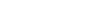# Running the 0-10V DAC I2C with a Raspberry Pi

Hi there. I have a 0-10V DAC I2C (https://store.ncd.io/product/1-channel-0-10v-dac-digital-analog-converter-i²c/). I have connected the DAC module to a Raspberry Pi 4 using a I2C shield. And I have I2C enabled on the Raspberry Pi. But, I couldn’t find any Python codes on the product website to run the DAC module. Do you have an example Python code that I can use that would work on a Raspberry Pi? Also, are there any libraries that I would need to install. Sorry, I’m very new to this. Thank you.

i use this sample code for testing

Hi Bhaskar, thank you for your code. In your code, which variable corresponds to the output voltage? For example, if I want to set the output voltage to say 6V, which variable would I change in your code? I’m guessing “i” is just an index. Is “data” the variable that I would need to change? Thanks!

data is the variable which needs to be changed in order to change the voltage output

Your code works correctly when I run it. I tried changing your code to send a single DAC value, 2048, which should correspond to 5 Volts. This is what I wrote:

import smbus
bus = smbus.SMBus(1)
data = [2048, 2048]
bus.write_i2c_block_data(0x60, 0x41, data)

However, I’m not getting the right voltage output. Do you know what I’m doing wrong here? Thanks.

You can use the code i shared as it is.

The DAC output will goo from 0V to 10V.
for i in range(0,4095):
the value of i will decide the voltage output.
Thanks

Hi, we need the DAC to output a constant voltage. We can’t have the DAC output incrementally increase from 0V to the setpoint. That would affect our experiment. Is there a code/command to send a single, unchanging output voltage to the DAC. Thanks.

https://wiki.python.org/moin/BitwiseOperators

x << y Returns x with the bits shifted to the left by y places
x >> y Returns x with the bits shifted to the right by y places
x & y Bitwise and

data1 and data2 are the Most Significant Byte (MSB) and Least Significant Byte (LSB).

easiest way to do is set the value of “i” between line 9 and 10.

for example if you want o set voltage output 5V then set the " i = 1024"

Thanks! That works. : )